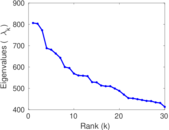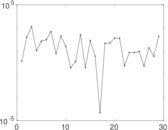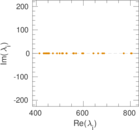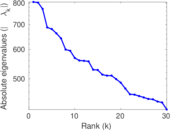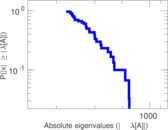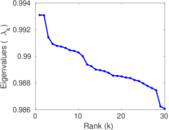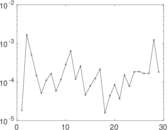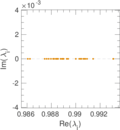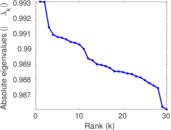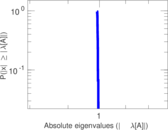This network consists of the wikilinks of the Wikipedia in the Chinese language (zh). Nodes are Wikipedia articles, and directed edges are wikilinks, i.e., hyperlinks within one wiki. In the wiki source, these are indicated with [[double brackets]]. Only pages in the article namespace are included.

 Code `Wzh` Internal name `wikipedia_link_zh` Name Wikipedia links (zh) Data source http://dumps.wikimedia.org/ AvailabilityDataset is available for download Consistency checkDataset passed all tests Category Hyperlink network Node meaning Article Edge meaning Wikilink Network format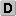Unipartite, directed Edge typeUnweighted, no multiple edges ReciprocalContains reciprocal edges Directed cyclesContains directed cycles Loops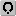Contains loops

## Statistics

 Size n = 1,786,381 Volume m = 72,614,837 Loop count l = 4,325 Wedge count s = 115,820,026,630 Claw count z = 2,249,126,503,739,186 Cross count x = 5.541 29 × 1019 Triangle count t = 2,728,052,766 Maximum degree dmax = 162,403 Maximum outdegree d+max = 4,286 Maximum indegree d−max = 162,402 Average degree d = 81.298 3 Size of LCC N = 1,786,182 Size of LSCC Ns = 1,219,833 Relative size of LSCC Nrs = 0.682 852 Diameter δ = 11 50-Percentile effective diameter δ0.5 = 3.247 45 90-Percentile effective diameter δ0.9 = 4.304 90 Median distance δM = 4 Mean distance δm = 3.736 55 Balanced inequality ratio P = 0.165 864 Outdegree balanced inequality ratio P+ = 0.169 982 Indegree balanced inequality ratio P− = 0.171 280 Degree assortativity ρ = −0.037 324 1 Degree assortativity p-value pρ = 0.000 00 Clustering coefficient c = 0.070 662 7 Directed clustering coefficient c± = 0.646 979 Operator 2-norm ν = 862.541 Cyclic eigenvalue π = 805.993 Reciprocity y = 0.570 321 Non-bipartivity bA = 0.491 122 Normalized non-bipartivity bN = 0.031 713 3

## Plots

### Degree distribution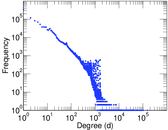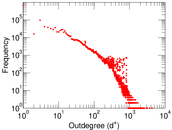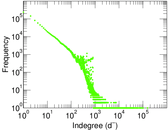### Cumulative degree distribution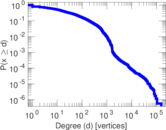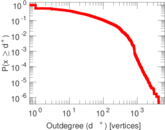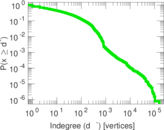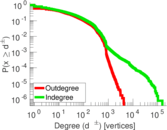### Hop distribution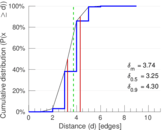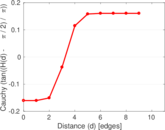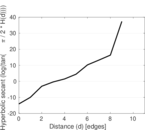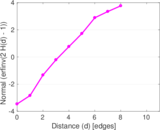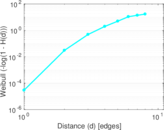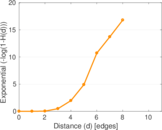### In/outdegree scatter plot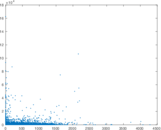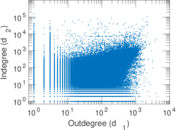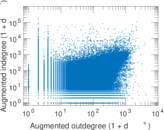### Matrix decompositions plots